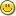### 1. countdown display code

The following code displays a countdown intended to update every second. Most often the seconds display a decrement of 2 seconds, sometimes a 1 second decrement is displayed.

Is there an error in my code?

```--
-- count_down.ex
--
include std/datetime.e
include std/math.e
include std/graphics.e
include std/os.e

function DaysBetweenDates(datetime dt1,datetime dt2)
atom d,h,m,s=diff(dt1,dt2)
d=s/86400
h=frac(d)*24 -- hours.xxx
d=trunc(d)   -- days
m=frac(h)*60    -- minutes.xxx
h=trunc(h)      -- hours
s=frac(m)*60   -- seconds.xxx
m=trunc(m)     -- minutes
s=trunc(s)    -- seconds
return {d,h,m,s}
end function

public procedure now_until(datetime dt2={2020,9,1,0,0,0})
sequence bal, pos = get_position()
loop do
text_color(BRIGHT_WHITE)
position(pos,pos) puts(1,"            ") position(pos,pos)
text_color(BLUE)
bal=DaysBetweenDates(now(),dt2)
printf(1,"%d-%d:%d:%d",{bal,bal,bal,bal})
sleep(1)
until equal(bal,dt2)
end loop
end procedure

now_until()
```

Thanks, Ken

### 2. Re: countdown display code

Not exactly an error, more a misunderstanding of how truncate works, and Euphoria's printf() hiding what's really happening. trunc() returns the integer portion of a number, so even 9.9999999999 will come out as 9. That's not what's needed here.

```--
-- count_down.ex (revised)
--
include std/datetime.e
include std/math.e
include std/graphics.e
include std/os.e
include std/console.e
atom s,t,r

function DaysBetweenDates(datetime dt1,datetime dt2)
atom d,h,m
s=diff(dt1,dt2)
d=s/86400
h=frac(d)*24 -- hours.xxx
d=trunc(d)   -- days
m=frac(h)*60    -- minutes.xxx
h=trunc(h)      -- hours
s=frac(m)*60   -- seconds.xxx
m=trunc(m)     -- minutes
t = trunc(s)
r = round(s,1)
return {d,h,m,s}
end function

public procedure now_until(datetime dt2={2020,9,1,0,0,0})
sequence bal, pos = get_position()
object prevbal = 0
loop do
bal=DaysBetweenDates(now(),dt2)
display("[]-[]:[]:[] ",bal,0) display("s=[] t=[] r=[]",{s,t,r})
sleep(1)
until equal(bal,dt2)
end loop
end procedure

now_until()

```

The actual numbers are like this: s = raw seconds, t = trunc(s), r = round(s)

```15-9:42:16 s=16 t=16 r=16
15-9:42:15 s=15 t=14 r=15
15-9:42:14 s=14 t=14 r=14
15-9:42:13 s=13 t=12 r=13
15-9:42:12 s=12 t=11 r=12
15-9:42:11 s=11 t=11 r=11
15-9:42:9.99999999999998 s=9.99999999999998 t=9 r=10
15-9:42:9.00000000000002 s=9.00000000000002 t=9 r=9
15-9:42:7.99999999999999 s=7.99999999999999 t=7 r=8
15-9:42:7.00000000000004 s=7.00000000000004 t=7 r=7
15-9:42:6 s=6 t=6 r=6
15-9:42:4.99999999999997 s=4.99999999999997 t=4 r=5
15-9:42:4.00000000000002 s=4.00000000000002 t=4 r=4
15-9:42:2.99999999999998 s=2.99999999999998 t=2 r=3
15-9:42:2.00000000000003 s=2.00000000000003 t=2 r=2
15-9:42:0.999999999999996 s=0.999999999999996 t=0 r=1
15-9:41:60 s=60 t=59 r=60
15-9:41:59 s=59 t=59 r=59
15-9:41:58 s=58 t=57 r=58

```

Note: Will you have to use round instead of trunc for the minutes, hours and days as well?

### 3. Re: countdown display code

Here's a version that avoids nasty floating point math:

```
include std/os.e
include std/console.e
include std/datetime.e

public procedure now_until(datetime dt={2021,12,25,12,0,0})
object dt1 -- (computer time and date)
object dt2 = to_unix(dt)
object target = datetime:format(dt,"%A, %b %d, %Y %l:%M:%S %p")
object diff -- in seconds
integer sec_now = 0, sec_prev = 0
loop do
dt1 = to_unix(datetime:now())
diff = dt2-dt1
diff = from_unix(diff)
diff -= {1970,1,1,0,0,0} -- adj for unix EPOCH
sec_now = diff
if sec_now != sec_prev then
display("Time remaining: [b]-[b]-[b] []:[]:[]",diff,0)
display(" until []",{target})
sec_prev = diff
end if
sleep(.1) -- note: must check more than once per second, or time will "jitter"
until equal(dt1,dt2)
end loop
end procedure

now_until()

```

Now you know how much time you have to buy me a Christmas gift for next year:

```Time remaining: 1-4-10 19:37:9 until Saturday, Dec 25, 2021 12:00:00 AM
Time remaining: 1-4-10 19:37:8 until Saturday, Dec 25, 2021 12:00:00 AM
Time remaining: 1-4-10 19:37:7 until Saturday, Dec 25, 2021 12:00:00 AM
```

### 4. Re: countdown display code

Super WOW! Many thanks Irv!

Oh, and thanks for all the neat Pastey demos too.Regards, Ken

### 5. Re: countdown display code

I revised Irv's 1st revision using round instead of trunc, display instead of printf and sleep(.1) instead of sleep(1) per his sample code.. works like charm.

Thanks, again,

```function DaysBetweenDates(datetime dt1,datetime dt2)
atom h,m,s=diff(dt1,dt2),
d=s/86400     -- days.xx
h=frac(d)*24 -- hours.xxx
d=round(d,1) -- days
m=frac(h)*60      -- minutes.xxx
h=round(h,1)      -- hours
s=frac(m)*60     -- seconds.xxx
m=round(m,1)     -- minutes
s=round(s,1)     -- seconds
return {d,h,m,s}
end function

public procedure now_until(datetime dt2={2021,1,20,0,0,0})
sequence bal, pos = get_position()
object prevbal = 0
loop do
text_color(BRIGHT_WHITE)
position(pos,pos)
puts(1,"              ")
position(pos,pos)
text_color(BLUE)
bal=DaysBetweenDates(now(),dt2)
display("[]-[]:[]:[] ",bal,0)
sleep(.1)
until equal(bal,dt2)
end loop
end procedure

now_until()

-- 155-3:4:27
```

### 6. Re: countdown display code

Good job but there is another way you could do it.

You see, the 0.9999 comes from repeating decimals which happens in binary as often as in base-10. You often get non-terminating decimal places after dividing.

You see 1/3 gives you a non-terminating decimal, but the computer will keep a limited set of decimals. Multiply this by 3 and you get 0.99999999. Now 1/86400 is a non-terminating decimal in both binary and decimal. So when you multiply the values you get this just shy of the correct value problem. If you avoid having this fraction in the first place, you avoid the problem.

```atom t = floor(diff(d1,d2)) -- total_seconds.  A whole number but might be too big for integer type.
integer s = remainder(t,60) -- the seconds part of the time 0..59 (always an integer!)
t = floor(t/60) -- now total minutes
integer m = remainder(t, 60) -- the minute part of the time  0..59
t = floor(t/60) -- now total hours
integer h = remainder(t,24)  -- hour part of the time.
t = floor(t/24) -- now total days
-- etc ....
```